Premium

# 3.NBT.A.2 Worksheets, Workbooks, Lesson Plans, and Games

#### CCSS.maths.CONTENT.3.NBT.A.2

:
"Fluently add and subtract within 1000 using strategies and algorithms based on place value, properties of operations, and/or the relationship between addition and subtraction."

Our Take:A third grader should be confident adding and subtracting within 1000. Most students will have internalized a variety of strategies that help them solve these types of problems.

These worksheets can help students practise this Common Core State Standards skill.

## Worksheets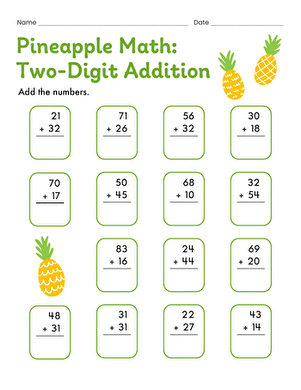Pineapple maths: Two-Digit Addition
Worksheet
Pineapple maths: Two-Digit Addition
Kids solve addition problems with two-digit addends and no regrouping on this year three maths worksheet.
Year 3
Maths
Worksheet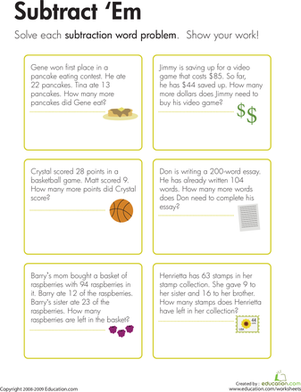Subtraction Word Problems: Subtract 'Em
Worksheet
Subtraction Word Problems: Subtract 'Em
Kids use details from the word problems on this year four maths worksheet to construct and solve subtraction problems with numbers up to three digits long.
Year 4
Maths
Worksheet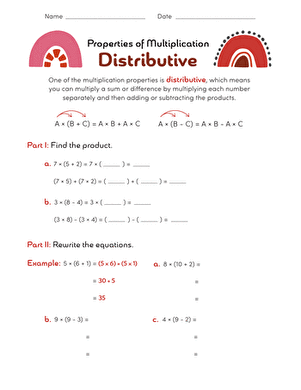Properties of Multiplication: Distributive
Worksheet
Properties of Multiplication: Distributive
Help your child wrap his head around the distributive property by challenging him to complete the equations in this worksheet according to this concept.
Year 4
Maths
Worksheet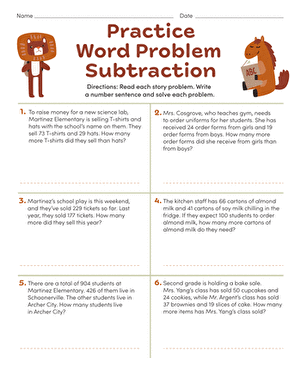Practise Word Problem Subtraction
Worksheet
Practise Word Problem Subtraction
Looking for a way to practise word problem subtraction? Get in two-digit and three-digit subtraction practise with simple word problems.
Year 4
Maths
WorksheetPractise Test: 3-Digit Addition & Subtraction
Worksheet
Practise Test: 3-Digit Addition & Subtraction
Review adding and subtracting three-digit numbers with this quick practise quiz.
Year 3
Maths
Worksheet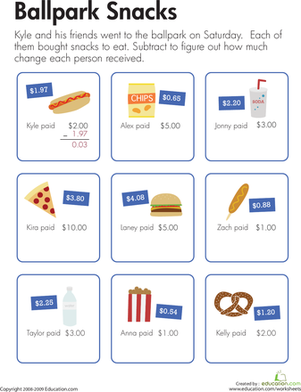Making Change at the Ballpark
Worksheet
Making Change at the Ballpark
On this year four maths worksheet, kids solve decimal subtraction problems as they calculate change on a trip to the ballpark.
Year 4
Maths
Worksheet

## Lesson PlansFluently Adding Within 1000
Lesson plan
Fluently Adding Within 1000
Adding big numbers can be tricky, but this lesson introduces your students to a strategy that makes it less intimidating! Using the place value strategy will make the task less daunting.
Year 4
Maths
Lesson planFluently Subtracting within 1000
Lesson plan
Fluently Subtracting within 1000
Start your students on subtracting three-digit numbers with this lesson that breaks the problems down by place values!
Year 4
Maths
Lesson planPlace Value: Digit Game
Lesson plan
Place Value: Digit Game
Help your students understand place values with this lesson that catches their interest using a story book. Students will go one to play a riddle game both in a group and individually.
Year 4
Maths
Lesson planSupply and Demand
Lesson plan
Supply and Demand
Do you want an interactive lesson that puts all the decision making in the palm of your students' hands? Supply and demand is a fun, interactive, and hands on activity that will help your students understand economics on a smaller scale.
Year 4
Maths
Lesson planBorrowing centre
Lesson plan
Borrowing centre
Using base ten blocks, cards, and clear cups, your students will practise the concept of borrowing for subtraction problems in this lesson.
Year 3
Maths
Lesson plan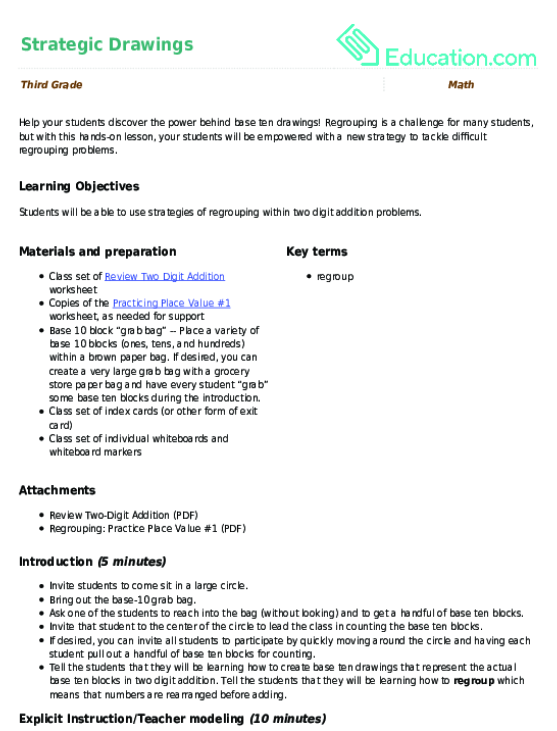Strategic Drawings
Lesson plan
Strategic Drawings
Help your students discover the power behind base ten drawings! Regrouping is a challenge for many students, but with this hands-on lesson, your students will be empowered with a new strategy to tackle difficult regrouping problems.
Year 4
Maths
Lesson plan

## Workbooks

No workbooks found for this common core node.

## GamesTwo- and Three- Digit Addition
Game
Two- and Three- Digit Addition
Kids solve two- and three-digit addition problems by regrouping in order to help the Professor grow out his beard.
Year 4
Maths
Game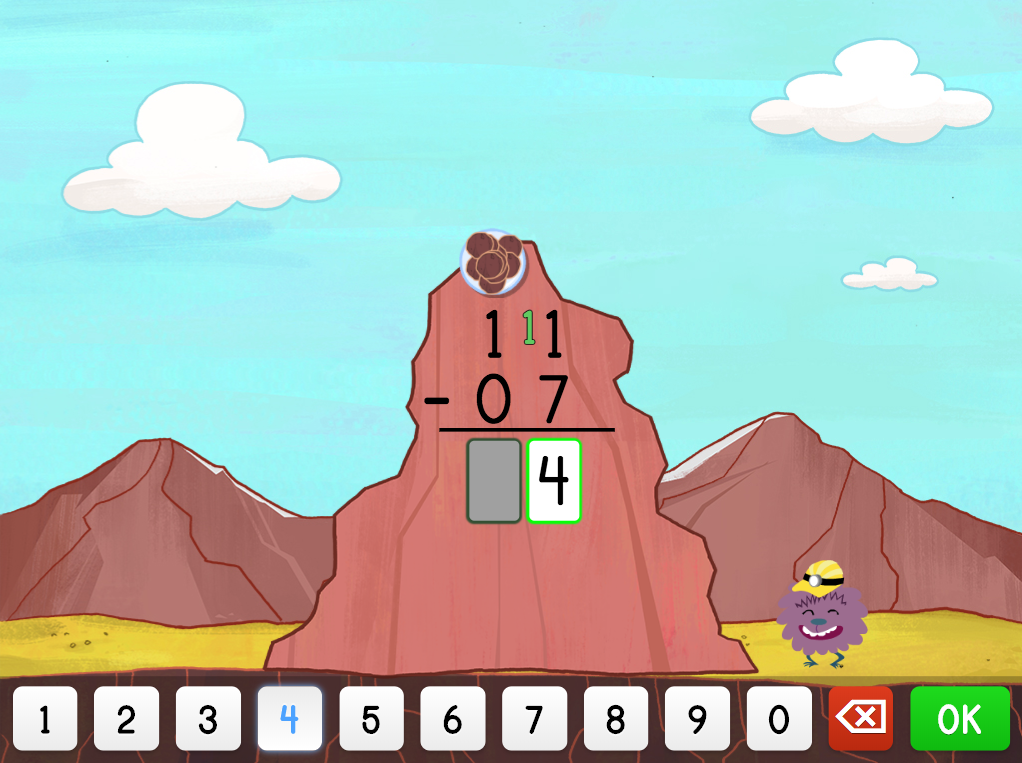Two-Digit Subtraction with Borrowing Mountain Game
Game
Two-Digit Subtraction with Borrowing Mountain Game
Kids practise borrowing in this two-digit subtraction mountain-themed game.
Year 4
Maths
Game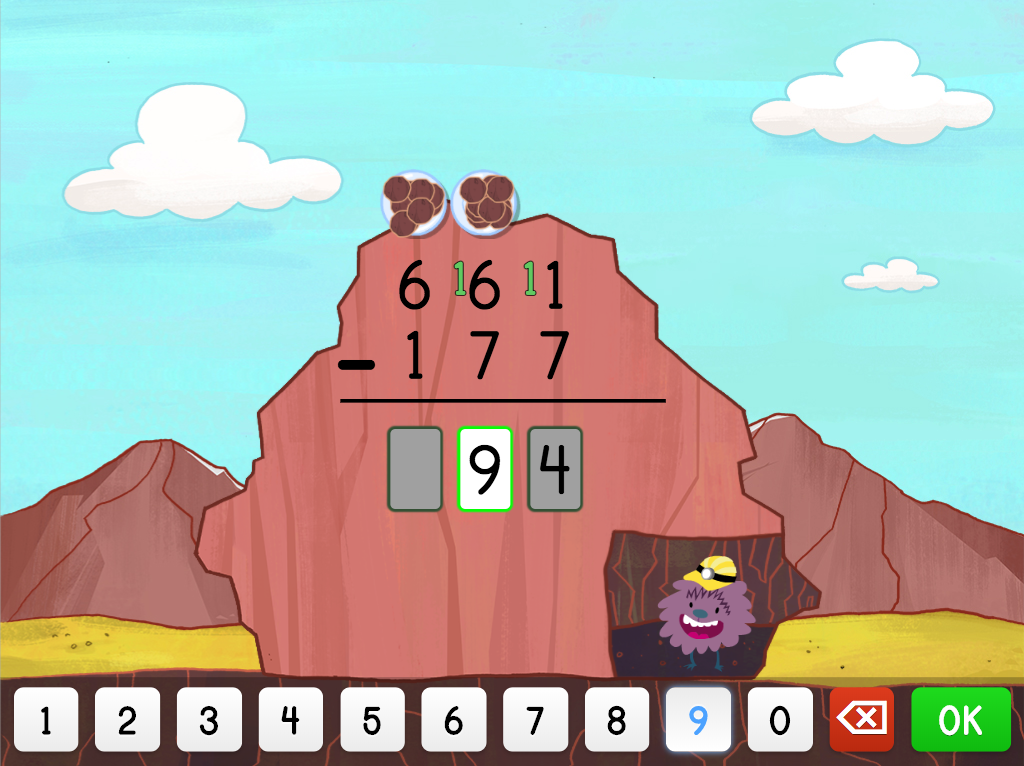Three-Digit Subtraction with Borrowing Mountain Game
Game
Three-Digit Subtraction with Borrowing Mountain Game
Kids practise borrowing in this three-digit subtraction mountain-themed game.
Year 4
Maths
Game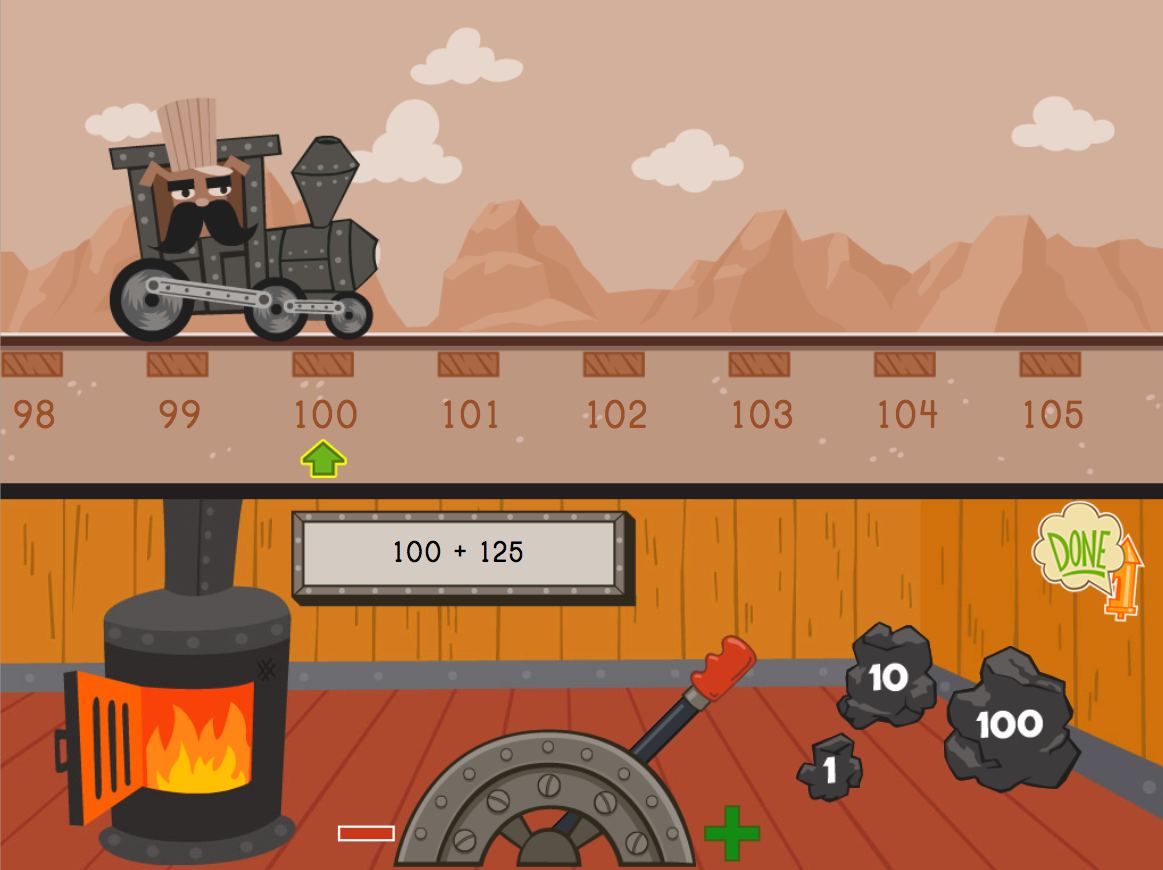Three-Digit Addition: Train Edition
Game
Three-Digit Addition: Train Edition
All aboard! Kids solve addition problems on a number line using 1, 10, and 100 unit manipulatives.
Year 4
Maths
Game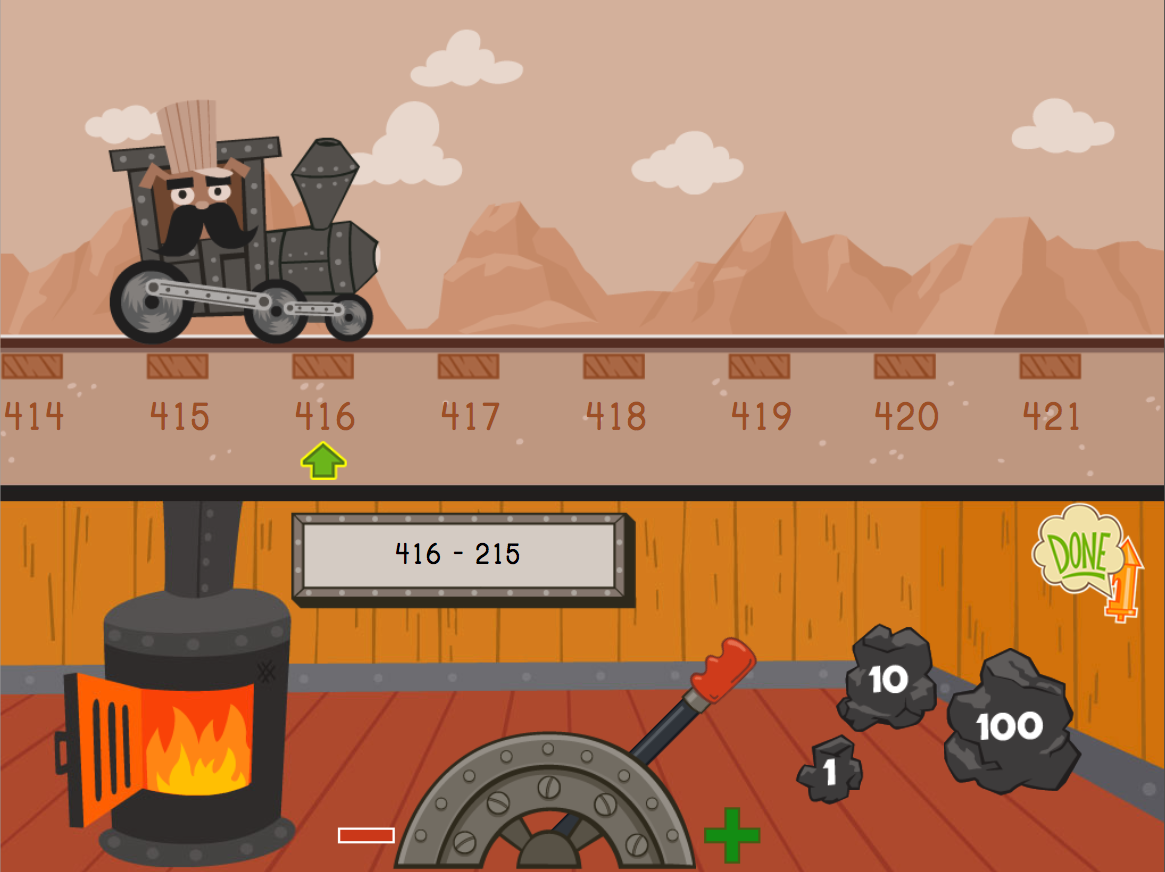Three-Digit Subtraction: Train Edition
Game
Three-Digit Subtraction: Train Edition
Choo-choo! Kids use a number line to solve three-digit subtraction problems in this fun-filled train game.
Year 4
Maths
Game

## ExercisesTwo-Digit Subtraction and Regrouping
Exercise
Two-Digit Subtraction and Regrouping
This exercise showing how to execute two digit subtraction using regrouping makes the second step in subtraction much more accessible.
Year 3
Maths
Exercise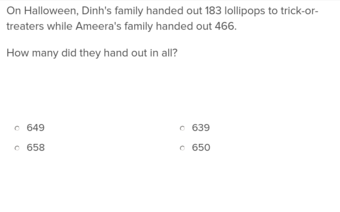Three-Digit Addition Word Problems
Exercise
Three-Digit Addition Word Problems
Teach students how to extract information from word problems and complete three digit addition to find the solution.
Year 4
Maths
Exercise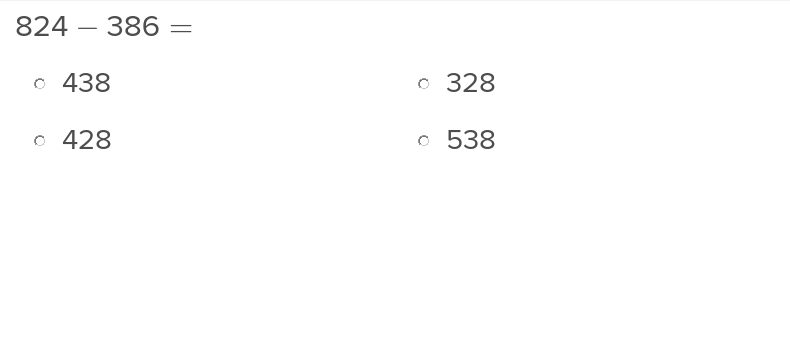Three-Digit Subtraction and Regrouping
Exercise
Three-Digit Subtraction and Regrouping
Teach students how to regroup, or borrow, during three digit subtraction problems with this easy to navigate exercise.
Year 4
Maths
Exercise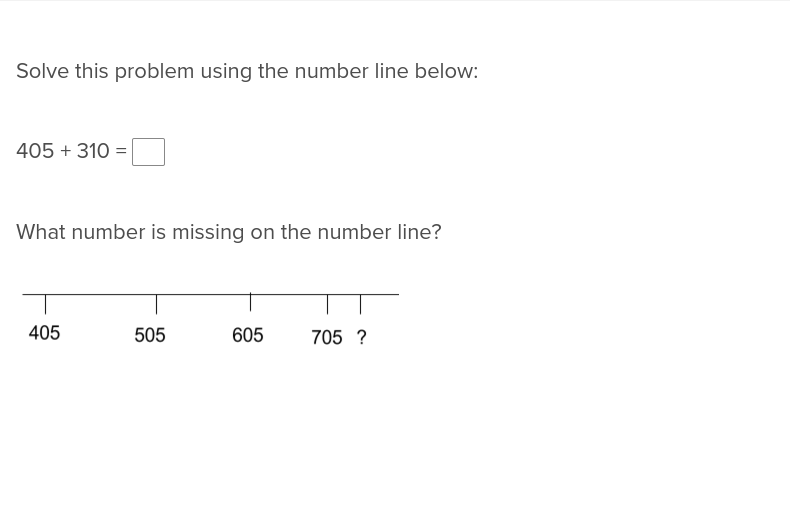Three-Digit Addition on a Number Line
Exercise
Three-Digit Addition on a Number Line
Simplify the seemingly complex task of three digit addition with the following number line activity.
Year 4
Maths
Exercise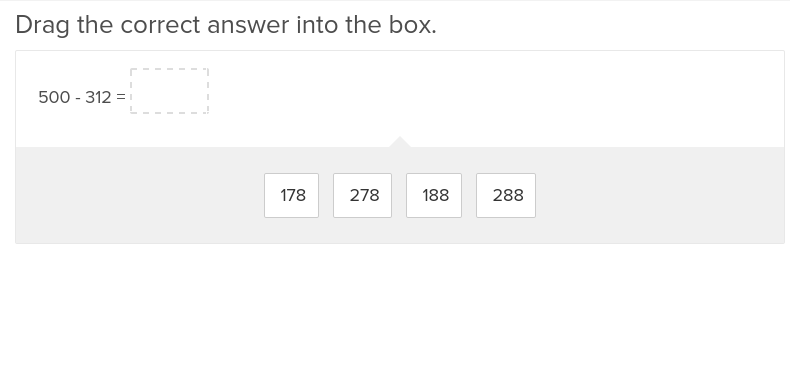Three-Digit Subtraction and Regrouping Numbers with Two Zeros
Exercise
Three-Digit Subtraction and Regrouping Numbers with Two Zeros
When students move on to three digit subtraction, they’ll appreciate the helping hand from this exercise that shows how to regroup numbers with two zeros.
Year 4
Maths
Exercise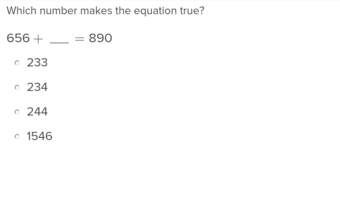Addition and Missing Factors
Exercise
Addition and Missing Factors
Adding up missing factors will be a routine matter for students after they complete this exercise.
Year 4
Maths
Exercise

### Add to collection

Create new collection

0

### New Collection>

0Items

What could we do to improve Education.com?

Please note: Use the Contact Us link at the bottom of our website for account-specific questions or issues.

What would make you love Education.com?